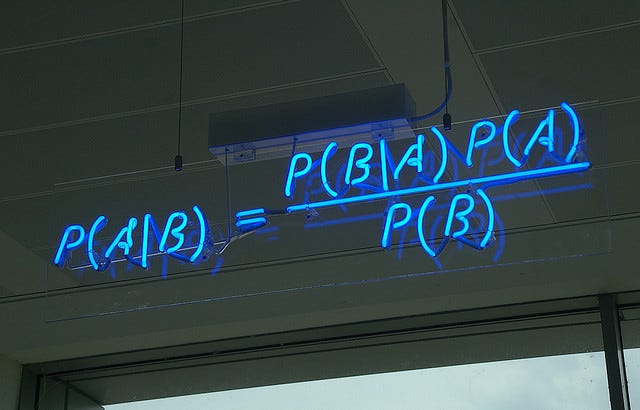# Naive Bayes From Scratch

In statistics, Naive Bayes classifiers are a family of simple “probabilistic classifiers” based on applying Bayes’ theorem with strong independence assumptions between the features. Source: WikipediaImage Source: Machine Learning Mastery

For the conceptual overview of Naive Bayes, refer — A Machine Learning Roadmap to Naive Bayes

We shall now go through the code walkthrough for the implementation of the Naive Bayes algorithm from scratch:

`import numpy as npclass NaiveBayes:    def fit(self, X, y):        n_samples, n_features = X.shape        self._classes = np.unique(y)        n_classes = len(self._classes)        # calculate mean, var, and prior for each class        self._mean = np.zeros((n_classes, n_features), dtype=np.float64)        self._var = np.zeros((n_classes, n_features), dtype=np.float64)        self._priors =  np.zeros(n_classes, dtype=np.float64)        for idx, c in enumerate(self._classes):            X_c = X[y==c]            self._mean[idx, :] = X_c.mean(axis=0)            self._var[idx, :] = X_c.var(axis=0)            self._priors[idx] = X_c.shape / float(n_samples)    def predict(self, X):        y_pred = [self._predict(x) for x in X]        return np.array(y_pred)    def _predict(self, x):        posteriors = []        # calculate posterior probability for each class        for idx, c in enumerate(self._classes):            prior = np.log(self._priors[idx])            posterior = np.sum(np.log(self._pdf(idx, x)))            posterior = prior + posterior            posteriors.append(posterior)                    # return class with highest posterior probability        return self._classes[np.argmax(posteriors)]                def _pdf(self, class_idx, x):        mean = self._mean[class_idx]        var = self._var[class_idx]        numerator = np.exp(- (x-mean)**2 / (2 * var))        denominator = np.sqrt(2 * np.pi * var)        return numerator / denominatorfrom sklearn.model_selection import train_test_splitfrom sklearn import datasetsdef accuracy(y_true, y_pred):    accuracy = np.sum(y_true == y_pred) / len(y_true)    return accuracyX, y = datasets.make_classification(n_samples=10000, n_features=10, n_classes=2, random_state=123) X_train, X_test, y_train, y_test = train_test_split(X, y, test_size=0.2, random_state=123)  nb = NaiveBayes() nb.fit(X_train, y_train) predictions = nb.predict(X_train)accuracy(y_train, predictions)Out:0.92025predictions = nb.predict(X_test)accuracy(y_test, predictions)Out:0.921`

Hope you enjoyed and made the most out of this article! Stay tuned for my upcoming blogs! Make sure to CLAP and FOLLOW if you find my content helpful/informative!

For complete code implementation:

## The Startup

Get smarter at building your thing. Follow to join The Startup’s +8 million monthly readers & +724K followers.

Written by

## Tanvi Penumudy

CS Undergrad at Bennett University## The Startup

Get smarter at building your thing. Follow to join The Startup’s +8 million monthly readers & +724K followers.

## More From Medium

Medium is an open platform where 170 million readers come to find insightful and dynamic thinking. Here, expert and undiscovered voices alike dive into the heart of any topic and bring new ideas to the surface. Learn more

Follow the writers, publications, and topics that matter to you, and you’ll see them on your homepage and in your inbox. Explore

If you have a story to tell, knowledge to share, or a perspective to offer — welcome home. It’s easy and free to post your thinking on any topic. Write on Medium